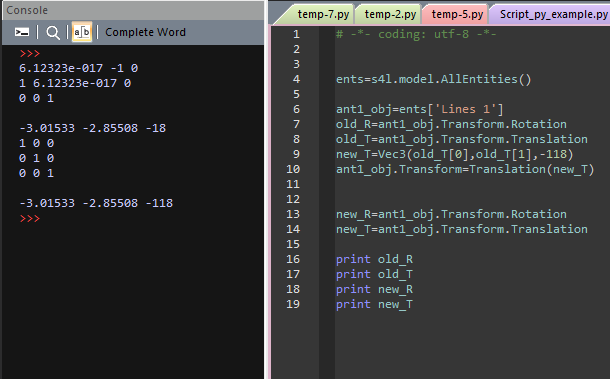# Entities Translation Transformation

I apply a Translational transformation to a group of entities without changing other parameters.
For the solid objects everything is fine, however for the Lines the Rotation property values are changed as well, even thought i dont apply any rotation. What might be the problem ?

It depends a bit on how you apply the transformation. Are you using the `ApplyTransform` function recursively to each of the entities of the group?
Can you add a screenshot of the translation/rotation properties of the Line object before and after you apply the translation?

@sylvain Below you can find the screenshot. I notice that the rotation matrix changes.
Also is there any way to input the old rotation matrix directly (without converting to an angle and a vector) ?The behavior you are describing is a related to a not-so-uncommon misunderstanding of the `.Transform` property.

Model entities in Sim4Life have a Transform property (which you access by `obj.Transform`, for example). This property holds the transformation matrix between the initial object and its final position. This means that it holds the composition of all the transformations that have been applied to this object since its creation. So when you write something like `obj.Transform = Rotation(axis, ori, ang)`, it does not just rotate the object - it replaces the transformation by a simple rotation, erasing all previous displacements.

To apply a rotation, one should instead use `obj.ApplyTransform(Rotation(axis, ori, ang))`. The same is true for applying a translation: first define a Translation object (which is usually independent of the Transform property of the object itself), then apply the transformation.

For example:

``````
from s4l_v1.model import Vec3, Translation, Transform
import numpy

t = Translation(Vec3(-20, -16, 10))           # for a translation
r = Rotation(Vec3(0, 0, 1), numpy.pi / 4)  # for a rotation of angle pi/4 around the z-axis

case = model.CreateSolidBlock(Vec3(0, 0, 0), Vec3(40, 16, -140))
case.ApplyTransform( t )
case.ApplyTransform( r )
``````